# Determine the force in each member of the roof truss shown. State whether each member is in tension or compression.

Question-AnswerCategory: Engineering MechanicsDetermine the force in each member of the roof truss shown. State whether each member is in tension or compression.

Determine the force in members AD. CD. and CE of the truss shown.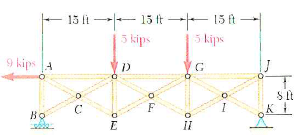Step: 1

Free body diagram of the truss is as shown.Step: 2

Let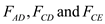be the forces in the members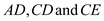respectively
Consider algebraic sum of moments about point K to be zero.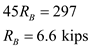Step: 3

Consider the algebraic sum of forces along the horizontal direction to be zero.Consider the algebraic sum of forces along the vertical direction to be zero.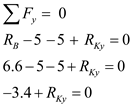Step: 4

Cut the truss through the membersto analyze the forces in them.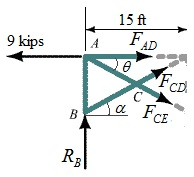Step: 5Consider triangle BDE.Step: 6

Consider algebraic sum of moments about point D to be zero.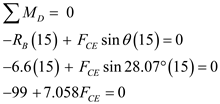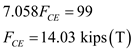Therefore, force in the member CE is.

Step: 7

Taking condition of equilibrium along the vertical direction,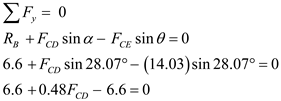Step: 8

Therefore, the force in the member CD is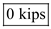.

Step: 9

Taking condition of equilibrium along the horizontal direction,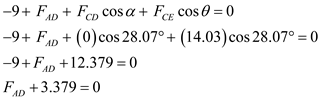Therefore, force in the member AD is.